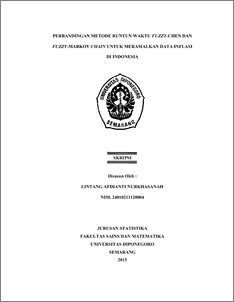# PERBANDINGAN METODE RUNTUN WAKTU FUZZY-CHEN DAN FUZZY-MARKOV CHAIN UNTUK MERAMALKAN DATA INFLASI DI INDONESIA

NURKHASANAH, LINTANG AFDIANTI (2015) PERBANDINGAN METODE RUNTUN WAKTU FUZZY-CHEN DAN FUZZY-MARKOV CHAIN UNTUK MERAMALKAN DATA INFLASI DI INDONESIA. Undergraduate thesis, FSM Universitas Diponegoro.Preview
PDF
3148Kb

## Abstract

Inflation data are financial time series data which often violate assumption if it is modeled with ARIMA Box-Jenkins classic method. Therefore, to forecast inflation are used forecast method which hasn’t requirement classic assumptions, like as fuzzy time series method. Fuzzy time series is a method of predicting data that use principles of fuzzy as basis. Many researches has been developed about this method, such as fuzzy time series developed by Chen (1996) and fuzzy time series-Markov chain developed by Tsaur (2012). In this case, both methods are used to predict inflation data in Indonesia. Result of predicting from both methods are compared with MSE value to in sample data. Method of fuzzy time series-Chen get MSE value 0,656, whereas method of fuzzy time series-Markov chain get MSE value 0,216. Because of this reason, method of fuzzy time series-Markov chain get smallest MSE value. So, this method as the best method. Furthermore, to evaluate the best of predicting model used MAPE value to out sample data. The MAPE value in method of fuzzy time series-Markov chain is 6,610%. As conclusion, model of fuzzy time series Markov chain have best performance. Keywords : Fuzzy time series, Markov chain , MSE, MAPE.

Item Type: Thesis (Undergraduate) H Social Sciences > HA Statistics Faculty of Science and Mathematics > Department of Statistics 47305 Mr Hasbi Yasin 05 Jan 2016 17:42 05 Jan 2016 17:42

Repository Staff Only: item control page